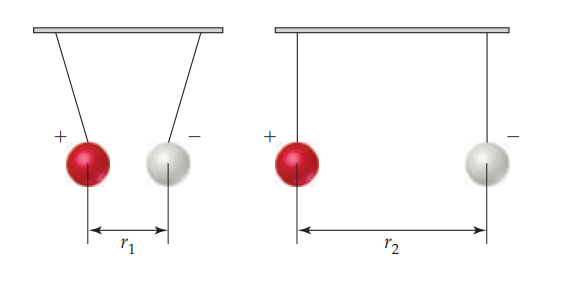×
Get Full Access to Chemistry: The Central Science - 14 Edition - Chapter 5 - Problem 5.28
Get Full Access to Chemistry: The Central Science - 14 Edition - Chapter 5 - Problem 5.28

×

# ?Consider a system consisting of two oppositely charged spheres hanging by strings and separated by a distance $$r_{1}$$, as shown in the accompanyingISBN: 9780134414232 1274

## Solution for problem 5.28 Chapter 5

Chemistry: The Central Science | 14th Edition

• Textbook Solutions
• 2901 Step-by-step solutions solved by professors and subject experts
• Get 24/7 help from StudySoup virtual teaching assistantsChemistry: The Central Science | 14th Edition

4 5 1 423 Reviews
10
5
Problem 5.28

Consider a system consisting of two oppositely charged spheres hanging by strings and separated by a distance $$r_{1}$$, as shown in the accompanying illustration. Suppose they are separated to a larger distance $$r_{2}$$, by moving them apart.

(a) What change, if any, has occurred in the potential energy of the system?

(b) What effect, if any, does this process have on the value of $$\Delta E$$?

(c) What can you say about q and w for this process?Text Transcription:

r_1

R_2

\Delta E

Step-by-Step Solution:

Step 1 of 5) (a) What change, if any, has occurred in the potential energy of the system(b) What effect, if any, does this process have on the value of ΔE(c) What can you say about q and w for this processLet’s use the periodic table to write the electron configuration of selenium (Se, element 34). We first locate Se in the table and then move backward from it through the table, from element 34 to 33 to 32, and so forth, until we come to the noble gas that precedes Se. In this case, the noble gas is argon, Ar, element 18. Thus, the noble-gas core for Se is [Ar]. Our next step is to write symbols for the outer electrons. We do this by moving across period 4 from K, the element following Ar, to Se:We begin with the 4s electrons, meaning our first two outer electrons are written 4s2. We then move into the d block, which begins with the 3d electrons. (The principal quantum number in the d block is always one less than that of the preceding elements in the s block, as seen in Figure 6.30.) Traversing the d block adds ten electrons, 3d10. Finally, we move into the p block, whose principal quantum number is always the same as that of the s block. Counting the squares as we move across the p block to Se tells us that we need four electrons, 4p4. The electron configuration for Se is therefore 3Ar44s23d104p4. This configuration can also be written with the subshells arranged in order of increasing principal quantum number: 3Ar43d104s24p4. As a check, we add the number of electrons in the [Ar] core, 18, to the number of electrons we added to the 4s, 3d, and 4p subshells. This sum s

Step 2 of 1

##### ISBN: 9780134414232

This full solution covers the following key subjects: . This expansive textbook survival guide covers 29 chapters, and 2820 solutions. Since the solution to 5.28 from 5 chapter was answered, more than 232 students have viewed the full step-by-step answer. The answer to “?Consider a system consisting of two oppositely charged spheres hanging by strings and separated by a distance $$r_{1}$$, as shown in the accompanying illustration. Suppose they are separated to a larger distance $$r_{2}$$, by moving them apart. (a) What change, if any, has occurred in the potential energy of the system? (b) What effect, if any, does this process have on the value of $$\Delta E$$? (c) What can you say about q and w for this process? Text Transcription:r_1R_2\Delta E” is broken down into a number of easy to follow steps, and 81 words. Chemistry: The Central Science was written by and is associated to the ISBN: 9780134414232. This textbook survival guide was created for the textbook: Chemistry: The Central Science, edition: 14. The full step-by-step solution to problem: 5.28 from chapter: 5 was answered by , our top Chemistry solution expert on 10/03/18, 06:29PM.

## Discover and learn what students are asking

Calculus: Early Transcendental Functions : Multiple Integration
?In Exercises 1 and 2, evaluate the integral. $$\int_{y}^{2 y}\left(x^{2}+y^{2}\right) d x$$

Statistics: Informed Decisions Using Data : Comparing Three or More Means (One-Way Analysis of Variance)
?Soybean Yield The data in the table represent the number of pods on a random sample of soybean plants for various plot types. An agricultural research

Statistics: Informed Decisions Using Data : Inference about the Difference between Two Medians: Dependent Samples
?Rats’ Red Blood Cell Counts Researchers at NASA wanted to learn the effects of spaceflight on a rat’s red blood cell count (RBC). The following data r

Unlock Textbook Solution

?Consider a system consisting of two oppositely charged spheres hanging by strings and separated by a distance $$r_{1}$$, as shown in the accompanying